Showing posts with label Inverse Square Law. Show all posts
Showing posts with label Inverse Square Law. Show all posts

## Wednesday, April 09, 2014

### Why are Planets Round?

Learn how the force of gravity influences the shapes of planets. Visit a gravity trap and learn about the ultimate gravity trap, a black hole.

***

Schumann Resonances

So one might look at the earth in an interesting way other then in a gravitational one.

Lithospheric Magnetic Anomalies. A modeled image of Earth's magnetic field variations created as a result of science satellites like Magsat. nT = nanoteslas. The color bar indicates areas with positive and negative magnetic fields.

See: Space Geodesy Project

***

## Thursday, May 07, 2009

### Defining the Space your Living In

The general theory of relativity is as yet incomplete insofar as it has been able to apply the general principle of relativity satisfactorily only to gravitational fields, but not to the total field. We do not yet know with certainty by what mathematical mechanism the total field in space is to be described and what the general invariant laws are to which this total field is subject. One thing, however, seems certain: namely, that the general principal of relativity will prove a necessary and effective tool for the solution of the problem for the total field.Out of My Later Years, Pg 48, Albert Einstein

Because Albert Einstein ended his career there, it did not mean such progressiveness would not move forward to include such an attempt to consider the "total field." By definition and allocation of a "step off point" people began to consider this possibility and sought such reformation in thinking as well

It is always the effort to see that such progressions in thought could have transformed any thinking person by the laws and rule of measure that will hold perspective toward the future. These are always being redefined by experiment, and such validations are adjusted then, to what we now use them for.

A new way to measure climate? A gravitational perspective held by Grace?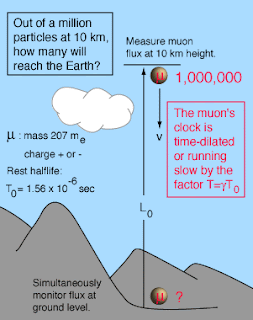The calculation will be considered from the Earth frame of reference. The length is then unaffected since it is in the Earth frame. The halflife is in the muon frame, so must be considered to be time dilated in the Earth frame. You may substitute values for the height and the muon speed in the calculation below
See:Muon Experiment in Relativity

While one may use this knowledge then with an attempt to discover new vaults of "time in measure" and recorded for historical pursuance by civilizations hidden in the pyramids, such efforts revealed nothing. They were not thinking the right way. It is the model developmental aspect which I have demonstrated over and over again that we can conceal the history of memories under such a analogical tool for pathways in the human sphere of perspective?Illustrations: Sandbox Studio See:Secrets of the Pyramids By Haley Bridger Symmetry Magazine

***

Such dynamical thinking then is the realization that our views had been transformed from straight lines and such to geometric that help us to think dynamically in the world around us.The Friedmann equation which models the expanding universe has a parameter k called the curvature parameter which is indicative of the rate of expansion and whether or not that expansion rate is increasing or decreasing. If k=0 then the density is equal to a critical value at which the universe will expand forever at a decreasing rate. This is often referred to as the Einstein-de Sitter universe in recognition of their work in modeling it. This k=0 condition can be used to express the critical density in terms of the present value of the Hubble parameter.

For k>0 the density is high enough that the gravitational attraction will eventually stop the expansion and it will collapse backward to a "big crunch". This kind of universe is described as being a closed universe, or a gravitationally bound universe. For k<0 the universe expands forever, there not being sufficient density for gravitational attraction to stop the expansion.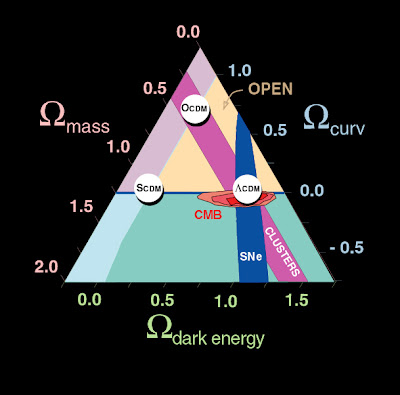Friedman Equation What is pdensity.

What are the three models of geometry? k=-1, K=0, k+1

Negative curvature

Omega=the actual density to the critical density

If we triangulate Omega, the universe in which we are in, Omegam(mass)+ Omega(a vacuum), what position geometrically, would our universe hold from the coordinates given?

If such a progression is understood in the evolution of the geometry raised in non euclidean perspectives, this has in my view raised the stakes on how we perceive the dynamical valuation of a world that we were lead into from GR?

***

"In a sentence, the observations are spectacular and the conclusions are stunning," said Brian Greene of Columbia University in New York City. "WMAP data support the notion that galaxies are nothing but quantum mechanics writ large across the sky." "To me, this is one of the marvels of the modern scientific age."

Such dynamics then are not just held to what we see of the earth frame but of what we hold in terms of our cosmological recognition of those same dynamics. How much sand then when reductionism has run it's limit that we say how far our perspective has gone into the powers of ten, that we see a limit had been reached?

This pursuance did not in the reductionist point of view reduce our apprehension of the world around us but we engaged the world to see that such length contractions are still vital measures for perspective.

IN the "mean time," we live in a vary dynamical world. If dark energy or dark matter seemed unrealistic then what measure of that space shall you consider to be, and that it shall not be, in the relationship of "time variable moments?"

IN the space of our cosmos we saw a satellite measure say that the expansion is speeding up/slowing down? Does this take away the dynamics of non euclidean geometries to say that this is an abstract version of math of what does not exist?Here L is called the Lagrangian. In simple cases the Lagrangian is equal to the difference between the kinetic energy T and the potential energy V, that is, L = T – V. In this interactive document we will approximate a continuous worldline with a worldline made of straight connected segments. The computer then multiplies the value of (T – V) on each segment by the time lapse t for that segment and adds up the result for all segments, giving us an approximate value for the action S along the entire worldline. Our task is then to move the connected segments of the worldline so that they result in the minimum total value of the action S.

While such a explanation had been served by understanding how one can rescue another and how two different people can get their quicker is the idea that such a plan is possible in the recognition of the cosmos as well. You just had to learn to see inan dynamical way as well.

The Lagrange Points

The easiest way to see how Lagrange made his discovery is to adopt a frame of reference that rotates with the system. The forces exerted on a body at rest in this frame can be derived from an effective potential in much the same way that wind speeds can be inferred from a weather map. The forces are strongest when the contours of the effective potential are closest together and weakest when the contours are far apart.....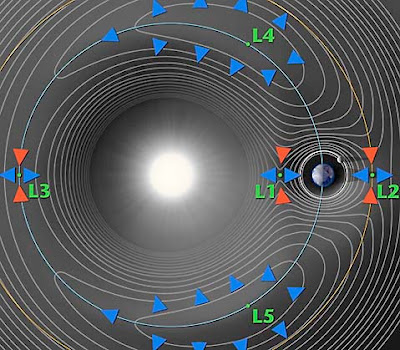A contour plot of the effective potential (not drawn to scale!).

In the above contour plot we see that L4 and L5 correspond to hilltops and L1, L2 and L3 correspond to saddles (i.e. points where the potential is curving up in one direction and down in the other). This suggests that satellites placed at the Lagrange points will have a tendency to wander off (try sitting a marble on top of a watermelon or on top of a real saddle and you get the idea). A detailed analysis (PDF link) confirms our expectations for L1, L2 and L3, but not for L4 and L5. When a satellite parked at L4 or L5 starts to roll off the hill it picks up speed. At this point the Coriolis force comes into play - the same force that causes hurricanes to spin up on the earth - and sends the satellite into a stable orbit around the Lagrange point.

The geometries as a whole seen in a local region, is the rule of law, as we move outward in space, or how else could we consider the dynamical movement that least resistance can fuel a path traveled with the least amount of energy expended?

***

See:
• Non Euclidean Geometry and the Universe
• Principal of Least Action
• Gran Sasso and the Pyramid
• ## Friday, October 03, 2008

### Centroids

Conclusion:The state of mind of the observer plays a crucial role in the perception of time.Einstein

It is often I consider the Center of Gravity(Mass) as an illustration of the perspective that exists "around and within us" as a product of the natural world. We are limited by our own knowledge, yet when given, and lead to observation of what is natural in our world, we see where we had not seen before.

\bar z = \frac{\int z dm}{m}The center of gravity (or mass), abbreviated as COM, of any object is that point within the object upon which gravity (or any body force) acts, regardless of the orientation of the object. The COM of an object may be calculated by using the principle of equilibrium.

First off you must know that I work according to a set of principles that were discovered after a time through "subjective reflection" on the nature of mind and how it works. Of course I am no expert here, but the principles themself are realizable as an active function in the recognition of how one might interpret the world.

\bar y = \frac{\int y \rho dV}{\int \rho dV}In the event that the density ρ of an object is not uniform throughout, the calculation of COM may be done by a similar set of equations involving the addition of density to the analysis.

It is by taking one to recognisance of the title of this blog entry that I wanted to discuss the "subjective part" and the understanding of the "mathematical construct" that one, in my opinion exists. Just as we progress to understand the natural world around us, I believe these are synonymous with each other.

\bar Y = \frac{\sum m \bar y}{\sum m}If a body is made up of multiple sections, each of which has a unique mass, the method for evaluating the centroid of that body is to evaluate the composite body by finite element analysis of each of the sections through the use of moment balancing, as above.

Liminocentric structuresYou can find much on this site in regards to this issue, and I now relate centroid for consideration in the case of this topic. I presented it at the Backreaction site today to show an advancement in the thinking as we move to incorporate the knowledge. As we come to understand the natural world around us.

Giving earth a new understanding in terms of its densities and it's relation to the gravitational contribution, it has in our new views of the globe, helped me learn and understand the feature of gravity as it extends to the cosmos.

I have taken it a step further as many of you know to include the emotive states as valued physiological relations to what manifests in the immediate environs of the body home. While this is a far cry from the understanding of gravitational research it was inevitable for me to see an extension of how one can topological relate to the world, in this inductive/deductive mode to reason.

While living on this earth, we always come home for reflection, and if any have not taken stock of what has accumulated during the day, then it is inevitable that is will come to reside very close to home as one sleeps through the nocturnality night. This research of subjectivity and mind had to entail "all of our activities." It had to include an understanding of the "mathematical constructs" that we observe, in order to relate to nature.

List of Centroids

ShapeFigure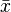$\bar x$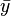$\bar y$Area
Triangular area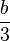$\frac{b}{3}$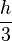$\frac{h}{3}$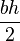$\frac{bh}{2}$
Quarter-circular area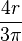$\frac{4r}{3\pi}$$\frac{4r}{3\pi}$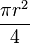$\frac{\pi r^2}{4}$
Semicircular area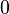$\,\!0$$\frac{4r}{3\pi}$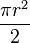$\frac{\pi r^2}{2}$
Quarter-elliptical area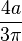$\frac{4a}{3\pi}$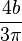$\frac{4b}{3\pi}$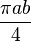$\frac{\pi a b}{4}$
Semielliptical areaThe area inside the ellipse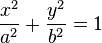$\frac{x^2}{a^2} + \frac{y^2}{b^2} = 1$ and above the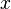$\,\!x$ axis$\,\!0$$\frac{4b}{3\pi}$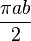$\frac{\pi a b}{2}$
Semiparabolic areaThe area between the curve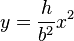$y = \frac{h}{b^2} x^2$ and the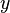$\,\!y$ axis, from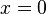$\,\!x = 0$ to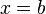$\,\!x = b$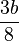$\frac{3b}{8}$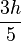$\frac{3h}{5}$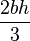$\frac{2bh}{3}$
Parabolic areaThe area between the curve$\,\!y = \frac{h}{b^2} x^2$ and the line$\,\!y = h$$\,\!0$$\frac{3h}{5}$$\frac{4bh}{3}$
Parabolic spandrelThe area between the curve$\,\!y = \frac{h}{b^2} x^2$ and the$\,\!x$ axis, from$\,\!x = 0$ to$\,\!x = b$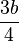$\frac{3b}{4}$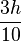$\frac{3h}{10}$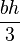$\frac{bh}{3}$
General spandrelThe area between the curve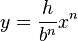$y = \frac{h}{b^n} x^n$ and the$\,\!x$ axis, from$\,\!x = 0$ to$\,\!x = b$$\frac{n + 1}{n + 2} b$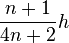$\frac{n + 1}{4n + 2} h$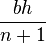$\frac{bh}{n + 1}$
Circular sectorThe area between the curve (in polar coordinates)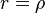$\,\!r = \rho$ and the pole, from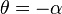$\,\!\theta = -\alpha$ to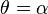$\,\!\theta = \alpha$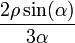$\frac{2\rho\sin(\alpha)}{3\alpha}$$\,\!0$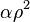$\,\!\alpha \rho^2$
Quarter-circular arcThe points on the circle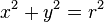$\,\!x^2 + y^2 = r^2$ and in the first quadrant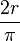$\frac{2r}{\pi}$$\frac{2r}{\pi}$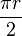$\frac{\pi r}{2}$
Semicircular arcThe points on the circle$\,\!x^2 + y^2 = r^2$ and above the$\,\!x$ axis$\,\!0$$\frac{2r}{\pi}$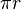$\,\!\pi r$
Arc of circleThe points on the curve (in polar coordinates)$\,\!r = \rho$, from$\,\!\theta = -\alpha$ to$\,\!\theta = \alpha$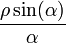$\frac{\rho\sin(\alpha)}{\alpha}$$\,\!0$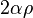$\,\!2\alpha \rho$

Why are Planets Round?

It is always interesting to see water in space.Image: NASA/JPL-
Planets are round because their gravitational field acts as though it originates from the center of the body and pulls everything toward it. With its large body and internal heating from radioactive elements, a planet behaves like a fluid, and over long periods of time succumbs to the gravitational pull from its center of gravity. The only way to get all the mass as close to planet's center of gravity as possible is to form a sphere. The technical name for this process is "isostatic adjustment."

With much smaller bodies, such as the 20-kilometer asteroids we have seen in recent spacecraft images, the gravitational pull is too weak to overcome the asteroid's mechanical strength. As a result, these bodies do not form spheres. Rather they maintain irregular, fragmentary shapes.

See:Isostatic Adjustment is Why Planets are Round?